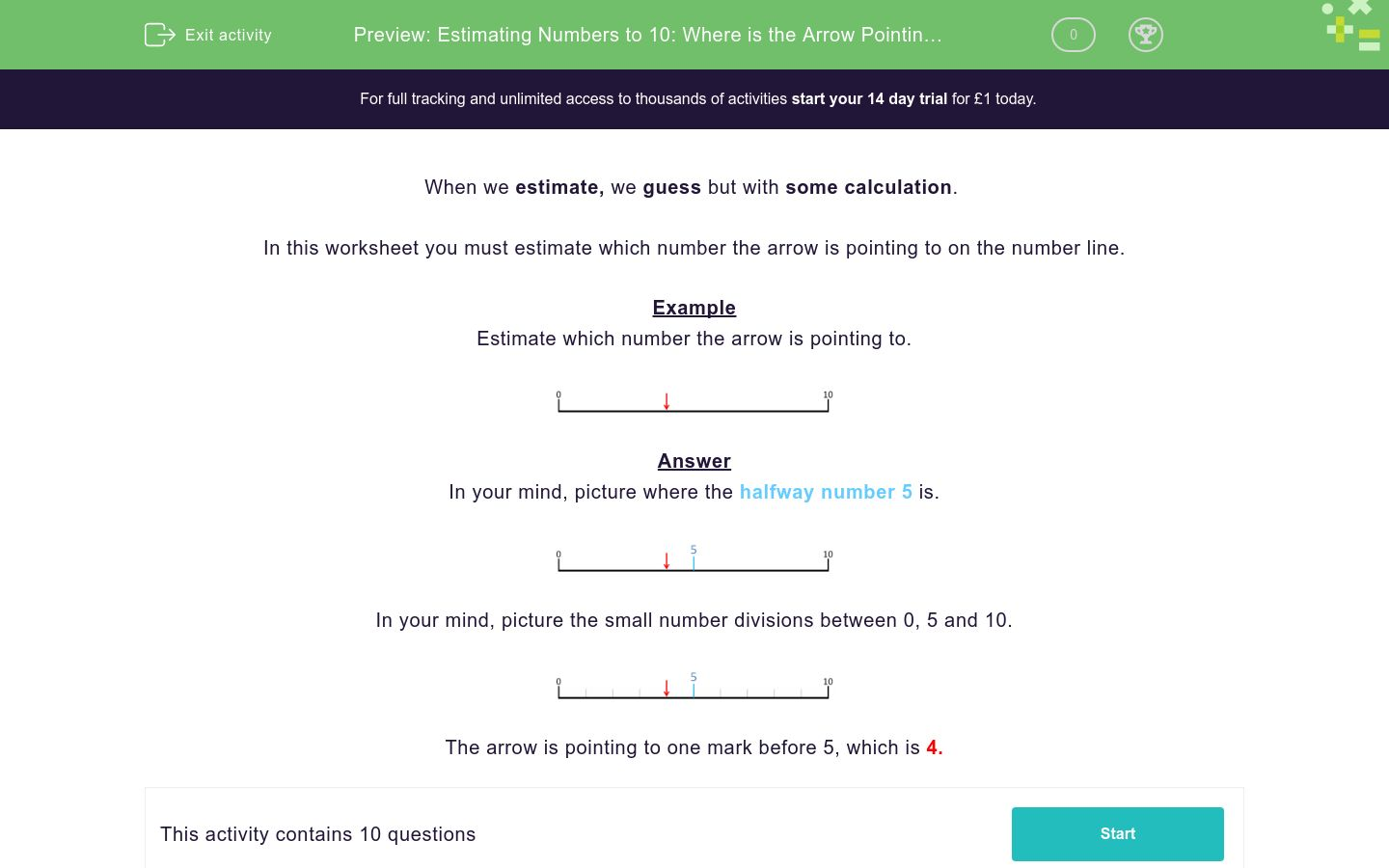Estimating Numbers to 10: Where is the Arrow Pointing? (1)

In this worksheet, students develop their estimation skills of numbers on a horizontal number line from 0 to 10.Key stage:  KS 1

Curriculum topic:  Number: Number and Place Value

Curriculum subtopic:  Represent Numbers to 100

Difficulty level:QUESTION 1 of 10

When we estimate, we guess but with some calculation

In this worksheet you must estimate which number the arrow is pointing to on the number line.

Example

Estimate which number the arrow is pointing to.In your mind, picture where the halfway number 5 is.In your mind, picture the small number divisions between 0, 5 and 10.The arrow is pointing to one mark before 5, which is 4.

Estimate which number the arrow is pointing to.Estimate which number the arrow is pointing to.Estimate which number the arrow is pointing to.Estimate which number the arrow is pointing to.Estimate which number the arrow is pointing to.Estimate which number the arrow is pointing to.Estimate which number the arrow is pointing to.Estimate which number the arrow is pointing to.Estimate which number the arrow is pointing to.Estimate which number the arrow is pointing to.• Question 1

Estimate which number the arrow is pointing to.10
EDDIE SAYS
The number 10 is shown!
• Question 2

Estimate which number the arrow is pointing to.1
EDDIE SAYS• Question 3

Estimate which number the arrow is pointing to.5
EDDIE SAYS• Question 4

Estimate which number the arrow is pointing to.4
EDDIE SAYS• Question 5

Estimate which number the arrow is pointing to.6
EDDIE SAYS• Question 6

Estimate which number the arrow is pointing to.9
EDDIE SAYS• Question 7

Estimate which number the arrow is pointing to.8
EDDIE SAYS• Question 8

Estimate which number the arrow is pointing to.2
EDDIE SAYS• Question 9

Estimate which number the arrow is pointing to.3
EDDIE SAYS• Question 10

Estimate which number the arrow is pointing to.7
EDDIE SAYS---- OR ----

Sign up for a £1 trial so you can track and measure your child's progress on this activity.

What is EdPlace?

We're your National Curriculum aligned online education content provider helping each child succeed in English, maths and science from year 1 to GCSE. With an EdPlace account you’ll be able to track and measure progress, helping each child achieve their best. We build confidence and attainment by personalising each child’s learning at a level that suits them.

Get startedStart your £1 trial today.
Subscribe from £10/month.